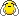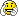# Variable problem

{
TFile f2(“ht.root”, “recreate”);
TTree *mytree = new TTree(“mytree”, “Test Tree”);
Double_t val = {0, 0, 0, 0, 0};
TBranch b2 = mytree->Branch(“b2”, val, “a/D:b:c:d:e”);
for (int i=0; i<8; i++) {
val = 1; val = -3.14; val = i
1.;
mytree->Fill() ;
}
f2.Write() ;
mytree->Print();
mytree->Scan();
}

please if a =7 and also a=3 how can i fill branch for this condition that’s when open branch a i will see 7 and 3```{ TFile f2("ht.root", "recreate"); TTree *mytree = new TTree("mytree", "Test Tree"); Double_t val = {0, 0, 0, 0, 0}; TBranch *b2 = mytree->Branch("b2", val, "a/D:b:c:d:e"); for (int i = 0; i < 8; i++) { val = i * 1.0 + 100.0; // set "a" val = i * 2.0 + 200.0; // set "b" val = i * 3.0 + 300.0; // set "c" val = i * 4.0 + 400.0; // set "d" val = i * 5.0 + 500.0; // set "e" mytree->Fill(); // fill "mytree" (will be entry number "i") } mytree->Write(); mytree->Print(); mytree->Scan(); }```

please i tried but not success
i have z=-1 and zz=1
how can i put the both in one branch Val that when i open Val i saw 1 and -1

pleaaaaaaaaaaaaaaaaaaaaase help me

The “val” (the “a” in “mytree”) is a single number (double-precision floating-point).
For each “mytree” entry, you can only have a single “a” value.

```// ... val = 1; mytree->Fill(); // ... val = -1; mytree->Fill(); // ...```thaaaaaaaaaaaaaaaaaaaaaank you too much=